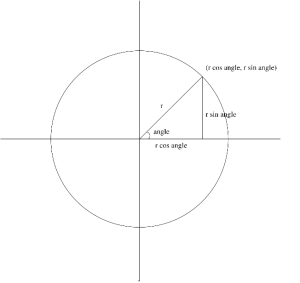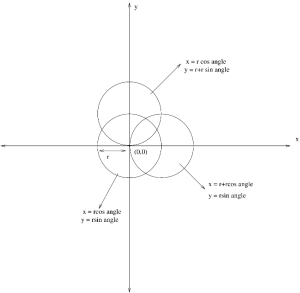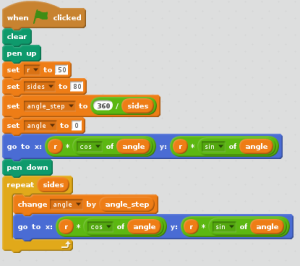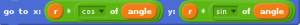## Circle Using Scratch

Polar Form of a Circle :
The polar coordinate system is a two-dimensional coordinate system in which each point on a plane is determined by a distance from a fixed point and an angle from a fixed direction. Keeping the radius as constant value(as the radius of the circle of the cant change), the angle keeps on varying until the circle is complete.Here, we have a circle with radius r.
Along the x- axis it is r cos angle and along the y-axis it is r sin angle.
x : r cos angle
y : r sin angle
Circle moved along the axis with radius r :Three circles are shown in the figure.
Each circle has a radius of r(defined by the user).
Center Circle :
The circle at the center has origin of (0,0) and by using the polar form method a simple circle can be drawn by defining the radius r and the angle.
x = r cos angle
y = r sin angle
Circle on the top :
The circle on the top is along y axis. The origin of this circle is at the edge of the center circle on the y axis. So,the equation of this circle is
x = r cos angle
y = r+r sin angle
Circle on the right :
The circle on the right is along x axis. The origin of this circle is at the edge of the center circle on the x axis. So,the equation of this circle is
x = r+r cos angle
y = r sin angle
Drawing a Circle in Scratch using the Trigonometric Formula :There are number ways to draw a circle in Scratch. Lets have a look at how to draw a circle using the Trigonometric formula(Polar form).
Simple way to make a circle is to use the Motion Scripts and to use the Move keys and angle (360 degree) etc..
Create Variables :
Example ,